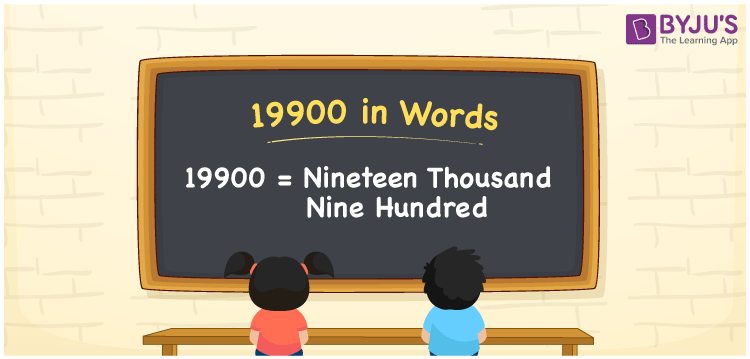# 19900 in Words

19900 in words is Nineteen Thousand Nine Hundred. If you earn Rs. 19900 per month, you can say, “I earn Rupees Nineteen Thousand Nine Hundred per month”. We know 19900 is a cardinal number since it represents a specific value. Here, we will learn to transform the number 19900 to word form using a place value chart.

 19900 in words Nineteen Thousand Nine Hundred Nineteen Thousand Nine Hundred in numerical form 19900

## 19900 in English Words

Generally, the English alphabet is used to write numbers in words. Thus, 19900 in English words is written as Nineteen Thousand Nine Hundred.## How to Write 19900 in Words?

The below chart shows the place value chart for the number 19900 which has five columns as 19900 has five digits.

 Ten-Thousands Thousands Hundreds Tens Ones 1 9 9 0 0

Hence, we can write the expanded form as:

1 x Ten Thousand + 9 x Thousand + 9 x Hundred + 0 × Ten + 0 × One

= 1 x 10000 + 9 x 1000 + 9 x 100 + 0 x 10 + 0 x 1

= 10000 + 9000 + 900 + 0 + 0

= 10000 + 9000 + 900

= 19900

= Nineteen Thousand Nine Hundred

Thus, 19900 in words is written as Nineteen Thousand Nine Hundred

Interesting way of writing 19900 in words

1 = One

19 = Nineteen

199 = One Hundred and Ninety-Nine

1990 = One Thousand Nine Ninety

19900 = Nineteen Thousand Nine Hundred

Hence, the word form of the number 19900 is Nineteen Thousand Nine Hundred

19900 is a natural number whose successor is 19901 and predecessor is 19899

• 19900 in words – Nineteen Thousand Nine Hundred
• Is 19900 an odd number? – No
• Is 19900 an even number? – Yes
• Is 19900 a perfect square number? – No
• Is 19900 a perfect cube number? – No
• Is 19900 a prime number? – No
• Is 19900 a composite number? – Yes

## Frequently Asked Questions on 19900 in Words

Q1

### Write 19900 in words.

19900 in words is written as Nineteen Thousand Nine Hundred.
Q2

### Simplify 18000 + 1900, and express in words.

18000 + 1900 = 19900 Thus, 19900 in words is Nineteen Thousand Nine Hundred.
Q3

### Is 19900 a composite number?

Yes, 19900 is a composite number.